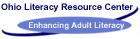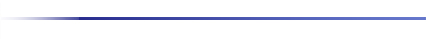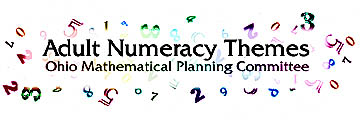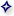You are here - OLRC Home   |   Site Index   |   Contact UsNumber and Number SenseNumber sense is a content skill that encompasses many areas in our adult education classroom. Number sense needs to be explored using whole numbers, fractions, decimals, percents, ratio, money, and estimation.

Being able to handle numbers comfortably and competently is important to adults as parents, workers, and community members. This competence relies upon having developed a kind of "number sense" about whole numbers, money, fractions, decimals, and percents. Number sense includes calculation skills with numbers as well as a sense of number and operation and an ability to appropriately use estimation, mental math, computation, calculators and other tools.

Computation skills are necessary but not sufficient. Adults must have strongly developed computational understanding of arithmetic numbers as well as some procedural knowledge of computation and number facts. They must be able to perceive the idea of place value and be able to read, write, and represent whole numbers and numerical relationships in a wide variety of way. Simple paper and pencil computations are not enough. Adults must be able to make decisions regarding the best method of computation to use in a particular situation; mental math, paper-and-pencil, calculator/computer. To demand individual paper and pencil computation from all students at all times does a disservice to our students and society. Problem solving skills are often best developed using a calculator and group work. Calculator work, mental math, and pencil-and-paper skills are important skills to develop in all students during all class time.

Even deeper to true number sense, are some very basic understanding such as sorting and classifying, comparing, ordering, counting and pattern recognition and development. There "pre-number and pre-operational" understandings apply to all numbers. To jump to computational skills without these concepts often spells disaster. For example, understanding basic fraction concepts is critical to making sense of the operations.

Operation sense or understanding how the four operations work means recognizing conditions in real world situation that indicate that the operation would be useful in those situations. Multiplicative reasoning is basic as it leads to the under-standing of multiplication, division, and proportional reasoning. This is the notion of "unitizing" or forming "units of units" where a person begins to groups objects together and consider them as sets or whole. Proportional reasoning is critical to people's ability to understand and communicate about (rather than compute) what averages and percents are. Anything that is decreasing or increasing or changing magnitude relies heavily on deep understanding of proportions, rates, ratios, relations and relative comparisons. These elements add to a dynamic definition of what we mean by being able to work with numbers.

Estimation and mental math are essential to sense making with numbers. Adults use estimation every day and all the time; it's woven into the fabric of daily decision making. Adults use estimation to predict and plan. It is used to check outcomes. Approximations guide thinking all along the way. It is a sense making activity. People need to be able to decide how precise they need to be in a particular situation.

Fractions, decimals, percents and ratios and their relationships with each other are basic skills. Fractions are often considered a hard topic from school. Yet these concepts are important for further understanding and exploration in math.

Knowledge of numbers is useful to adults in making decisions about issues that relate to their families, communities, and workplaces.

IMPLICATIONS FOR LEARNING AND TEACHING
Numbers must be taught and learned in context right from the beginning. Neatly controlled pages of computation are not the way adults learn best. If we expect life-long learning, we should use real-life problems. Learning how to compute percents in the context of a real life budget will be much more profitable than if taught in the abstract or with artificial work problems.

We must build on an adult's personal number sense. Traditional school math calculation methods are not always useful. The notion that adults should do it the "right way" or the teacher's way robs adults of their mathematical power. Good numeracy instruction must build upon an adult's personal number sense and help further develop that sense so that he/she can handle real life situation.

Adult educators must begin to question the teaching of "school math" especially when those strategies or techniques are rarely used by other competent adults. The way estimation is often taught, using precise rules for rounding, has nothing to do with the way people really use it in the workplace. The choice of teaching complicated fraction computation, which will never be used in real life must be weighed against more important and realistic skills.

Number sense enables adults to be able to interpret and represent the world in which they work and live. Good number sense supports the judgements and decisions that lead to independent action. Number sense is truly the cornerstone of mathematics. It is exemplified every day, whether we consider notions as complex as the consumer price index, as pivotal as the impact of the Great Depression, or as personal as a blood pressure reading.

ESTIMATION
There are times when we do not need to know the exact answer to a math problem. Sometimes an approximate answer will be all we need. People make mistakes in math and become of this they need a way to check their answers quickly. There are times when we will want to check our math to see if the answer we have arrived at is a sensible answer. Estimating is most useful in catching mistakes that are "way off" rather than mistakes that are close. It is important to know when an answer is reasonable, and so it is important to use estimation.

Estimation is an important part of the process of mathematical thinking. We need to provide time to predict, solve and reflect on our problem solving. Estimation and reasonableness are integral to prediction in a problem.

When you solve problems, you must first decide whether you need an exact answer or an estimate. Remember, even if problems require an exact answer, you can estimate to get the "ballpark" figure or after you complete your work in order to check the reasonableness of your answers.

Estimation is a critical life skill. Most often we use a calculator to solve problems. Being off by a factor of 10 will certainly change the answer even if the digits are correct. Usually in life, we do the "number crunching" with a calculator; we check the reasonableness of the answer using estimation. These are skills that need to be practiced in class.

Students need time to discuss instances when they have made estimates. Each instance can lead to a discussion of:

1. What the estimate sufficient or did you need an exact answer?
2. Was the answer reasonable?
Many times it makes sense to estimate. When estimating, remember that often a reasonable answer will not follow the rigid rule of "0-4 round down and 5-9 round up." Try to aim for number sense, rather than teaching rigid rules. To that end, endless drills rounding numbers to the nearest ten, hundreds, etc. are probably not beneficial for true number sense.

ESTIMATION IN PROBLEM SOLVING
ESTIMATING IS A SHORT CUT TO PROBLEM SOLVING WHEN AN EXACT ANSWER IS NOT NECESSARY OR WHEN CHECKING THE ACCURACY OF YOUR ANSWERS. ESTIMATING IS A PROCESS OF DETERMINING APPROXIMATE VALUES. BEING ABLE TO FIND AN APPROXIMATION IS USEFUL IN THREE WAYS:To find an approximate answer when an exact answer is not neededTo quickly check your results after you work a problem exactlyTo quickly pick the most reasonable answer from some answer choices given on some test questions

Suppose you have \$1.00. You choose three items at a grocery store that cost 41 cents, 29 cents, and 17 cents. To make sure you have enough money when you get to the check out counter follow the following steps;

41 ~ 40 Step 1- Round 41, 29, and 17 to the nearest ten
29 ~ 30 Step 2- Add 40, 30, and 20
17~ 20 Step 3- Compare 90 cents to the \$1.00 that you have
Adding 40, 30, and 20 is quicker and easier than adding 41, 29, and 17 which might require a pencil and paper or a calculator. The estimate you get is close and good enough to save you from embarrassing yourself at the checkout counter. 87 is what you get when adding 41, 29, and 17; 90 is a very good estimation of 87.

ESTIMATION IN MULTIPLICATION
Some multiplication problems can be done without long computation, once you know the right shortcuts.

 500 x 70 000 3500 35000 you can compute 70 X 500 this way.

7 X 5 = 35 here is a shortcut you can use to find the same product.
70
X 500
= 35000
###### 2+ 1 or 3 zero.

To estimate products, round each number. Then multiply the rounded numbers using the shortcut.

 estimate 37 X 54 37 ~ 40 4 x 5=20 54 ~ 50 40 x 50 = 2000 1 zero 1 zero since 37 x 54 = 1998, 2000 is a very good estimate

MULTIPLICATION BY 10, 100, OR 1,000.
To multiply by a number such as 40, 400, or 4,000, first multiply by 4, and then multiply by either 10, 100, or 1,000. A number such as 400 is a multiple of 100, since 4 times 100 equals 400.

For example, to multiply \$2.20 by 400:

1. multiply \$2.20 by 4. You-now have \$8.80.

2. now multiply by 100 by moving the decimal to places to the right in the first product, \$8.80. Now you have \$880, which is the product of 400x \$2.20.

MULTIPLYING NUMBERS THAT HAVE END ZEROS.
Sometimes either one of the factors, or both, have end zeros. Look at the problem Example A (140 x 2,000). To do this problem, you arrange and multiply only the numbers to the left of the end zeros. Then you attach to the products as many zeros as there are in both factors.

USING ESTIMATION IN WORD PROBLEMS
Estimation is especially useful in word problems.you can use estimation to help you decide which operation is correct for problem solving.you can use estimation to check the accuracy of your exact answer.

Example: Last Saturday, Peggy worked 5.75 hours of overtime. If her overtime salary rate is \$8.16 per hour, how much overtime did she earn that day?

An estimate can be quickly computed by replacing each number with a whole number and multiplying.

 5.75 ~ 6 8.16 ~ 8 6 x 8 = 48 The exact answer is \$46.92
Notice how 48 serves as a good clue:First, the amount 48 seems about right. This tells us that multiplication is the correct operation to use.Second, you know that the exact answer must be close to 48. If you compute an exact answer that is several dollars away from 48, you'll know that you probably made a mistake. You would then want to recheck your multiplication.

First estimate the answer and then compute by using your calculator.

1. If chicken is on sale for 1.04 per pound, how much would you pay for a whole chicken that weighs 5.95 pounds?

2. How much rock can Bryce carry with his truck in eight loads if his truck can carry 2 7\8 tons on each load?

ESTIMATION

 144 210 320 92 679 512 476 155 197 287

Work with a small group of students.

1) Without using pencil, paper or calculator, each person must pick three numbers from those shown in the chart to add to get a total as close to 1,000 as you can without going over.

2) Write your numbers on slips of paper. Then find an exact answer. Compare results with your group to determine who came the closest.

3) Discuss strategies for picking the numbers and list them. Did you estimate? What estimation method did you use? Why? Vary the activity by picking four numbers to get as close to 1,500 as you can.

Have students in each group create new estimation puzzles for their teammates to solve, using the numbers in the table. Suggest using subtraction as well as addition and limit number use to four numbers. Puzzles might take the form of two numbers whose difference is close to a specified numbers or adding two numbers and subtracting one number to get as close as possible to zero.

ESTIMATION IN GROUPS
OBJECTIVES:

Students will:
1. Learn estimating skills as related to shopping.

2. Demonstrate skills by developing a shopping list.

3. Develop interpersonal skills by group work.

4. Use addition, subtraction, and multiplication of decimals.

MATERIALS: Enough grocery store ad sheets so each group has one. Scenario cards.

GROUP ASSIGNMENT: There are many ways to assign groups. I normally stack the deck. I assign a confident student to each group initially until the students become confident in working in groups. With group experienced students I normally assign members at random. I never allow students to select. I ask the students to select a leader and to name their group. I then give each group a scenario card. I not only expect an answer but I ask the students to explain the process they used to arrive at the answer.

SCENARIO 1: ESTIMATION
You have \$35.00 and must buy enough groceries to feed your family of five for 6 meals. 1. Plan your meals based on the ad. 2. Estimate how much of each item you are buying. 3. You can not go over \$35 at the checkout. 4. The group that has the best menu and comes closest to \$35 without going over wins. REMEMBER;You are competing against the other groups, so keep the noise down.

SCENARIO 2: ESTIMATION
Your spouse gave you \$55.00 to buy supplies for a family picnic for 25 people, any money left over you can keep. By use of the ads,plan the picnic menu and estimate the cost. The group that arrives at the most realistic menu and cost closest to the estimation wins.

ESTIMATION IN GROUPS
OBJECTIVES:

Students will:
1. Learn estimation skills as related to attributes, fractions, decimals, percentages, and probability.

2. Demonstrate skills in projecting.

3. Develop interpersonal skills by group work.

4. Use math skills in conversion from decimal to fraction to percentages and back.

MATERIALS: 1 box Lucky Charms, Fruit Loops, or any other cereal with various colors and shapes.

SELECT GROUPS: 3-5 in a group. Random selection works best. Select a group leader and have the group select a name.

SCENARIO 1:
Place a pile of cereal on a table. Have each group look at the pile for a short period of time. On a separate piece of paper have the group estimate what fraction/percentage/decimal each color/shape is to the whole. As a class count out the colors and shapes, find the exact fraction/percentage/ decimal of the pile. The group closest wins. Show how charting would make the process easier.

SCENARIO 2:
Give each group a small pile of cereal. Without counting, have each group estimate the fraction/percent/decimal of colors and shapes. Compare with the actual figures. Which group came closest? Which had the most colors/shapes? What process was used?

ESTIMATION IN GROUPS (CEREAL CONT.)
OBJECTIVES:

Students will:
1. Demonstrate skills in graphing and proportion/ratio.

SCENARIO 3:
Give each group a pile of cereal. Have the group indicate the fraction/percentage/decimals of each shape/color in graph form. Encourage as many types of graphs as possible. Chart, line graph, pie chart, bar graph, pictograph. The group with the most accurate and varied wins.

SCENARIO 4:
Give each group a pile of cereal in two colors or shapes. Have each group arrive at a proportion/ratio of one color or shape to the other. On a sheet of paper have each group write an answer to the questions the teacher asks of all the groups. Questions should deal with the ratio if a certain number of colors were added or subtracted. What would the new number be? Estimate, then compute by cross multiplication. Stress that once the ratio is established it will not change just the numbers. Have each group member ask the questions, or one group.asking another.

ESTIMATION: Multiplying Decimals
Estimation is an essential skill to use when multiplying decimals. A lot of students are unsure or make mistakes in placing the decimal point. When multiplying with mixed decimals, the student should always multiply the whole numbers together to get the estimated answer and also the approximate decimal placement.

EXAMPLE: Bill unloaded 14 boxes that each weigh 2.1 pounds. How many pounds do the boxes weigh altogether?

 STEP 1 Estimate first. STEP 2 Line numbers up at right STEP 3 Multiply as with whole numbers STEP 4 Count the digits following each dec. point in the problem. From the right, count out this same number of decimal places in the answer. Insert dec. pt. 14 = 14 X 2.1~ 2 ~28 14 X 2.1 14 X 2.1 14 280 294 14 X 2.1 14 28 0 29.4 0 places +1 place
By using estimation one knows that the answer is going to be around 28 pounds. I was also sure that when I arrived at the answer of 29.4 pounds that the placement of the Decimal point was correct. If this were a multiple choice test I probably would not have to work the problem. The estimation would have been close enough to select the correct answer.

SOLVE THE FOLLOWING PROBLEMS BY ESTIMATION:

1.) 11.2 X 3.4
2.) 100.4 X 9.1
3.) 9.9 X 6.1
4.) 20.997 X 3.11
5.) \$11.01 X 6.19
6.) 89 X 10.2
7.) 3.9 X 4.1
8.) 12.3 X 2.1
9.) 6.9 X 3.1
10.) 199.8 X 1.99
11.) 9.78 X 3.97
12.) \$ 19.89 X 4.76

TITLE:
Planning a Budget/ Part 1 of 3, Calculating a Monthly Budget Income.

OBJECTIVES:
Students will understand the benefits of keeping a budget and will understand required math.

MATERIALS LIST:
Worksheets, lesson notes, calculators if desired.

TARGET AUDIENCE:
ABLE 5+ Math

INSTRUCTIONAL GROUP SIZE:
Small

ACTIVITY DESCRIPTION:
1. Discussing a budget.
2. Discussing a paycheck.
3. Calculating monthly income. III. Writing a Budget A. Vocab.

1 estimate - to guess. you can estimate the cost of an item.
2. luxuries - things you want but can live without.
3. necessities - things you must have
4. installment payments - bills that are paid in parts
5. upkeep - the cost of keeping something maintained
B. How
a. list necessities
b. list luxuries
c. include installment payments
d. include upkeep like keeping your car in good shape
e. remember both fixed and flexible expenses
f. out-of-pocket expenses - both luxuries and necessities
1. pocket change
2. cigarettes
3. coffee
4. pop
5. bus fare
6. lunch
C. When all expenses are subtracted from income, money should be left over. D. Complete worksheet luxuries/necessities E. Keeping a record of expenses - how?
l.Keep an actual record of all~money spent the first month. Prepare a budget using this information the second month.

2. There are expense forms which can be purchased, or make an individual one based on attached.

F. Complete worksheets

Luxuries or Necessities?
You may spend your pocket change on some of the following items. What items do you really need? Put an L for luxuries or an N for necessities. About how often do you buy these items in a month? Estimate the cost of each item. Multiply that by the number you buy each month to get the total spent on each item per month.

 L OR NCOSTAMOUNT BOUGHTTOTAL SPENTBUS FARE GUM CIGARETTES CANDY POP PIZZA BEER WINE ICE CREAM COFFEE CDs TAPES MAKEUP MOVIES BOOKS SCHOOL SUPPLIES SPORTS EVENTS BARS NIGHTCLUBS

MONTHLY BUDGET FORM

Income

Take home pay
Social Security/Other benefits
Other income
Total income

Monthly Expenses

Fixed
Rent/Mortgage Property tax Insurance Utilities Installment payments Car lease/payment Transportation Other
Total fixed

Monthly Expenses

Flexible
Groceries
Eating out/ordered in meals
Eating out snacks
House repairs
Gasoline
Car repairs
Clothing
Medical bills
Prescriptions
Cosmetics hair cuts
Laundry
School supplies
Newspapers
Movies/videos
Casettes/CDs
Recreation
Phone
Other
Total flexible

Set Aside Money

Savings
Contributions
Emergencies
Total Set Aside

Total of all ExpensesReturn to the TopThis page http://literacy.kent.edu/Oasis/Resc/Educ/numnum.html and is maintained by the OLRC .